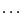Next: Kantorovitch theorem Up: Global implementation Previous: Global implementation   Contents

Example

An interesting example is given by:

Coeff_App(1)= INTERVAL(-3.1,-2.9);Coeff_App(2)=INTERVAL(1.9,2.1);
Coeff_App(3)=INTERVAL(-1.1,-0.9);Coeff_App(4)=INTERVAL(0.9,1.1);
Num=Global_Positive_Bound_Interval(3,Coeff_App,Lower,Upper);
The above procedure may give some sharp bounds. For example consider the Wilkinson polynomial of order 22 (which has as roots 1,2,,21,22) we get 0 negative roots while the positive roots are bounded by [0.790447,22.1087].